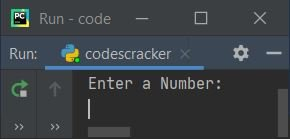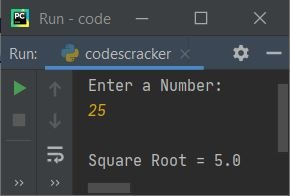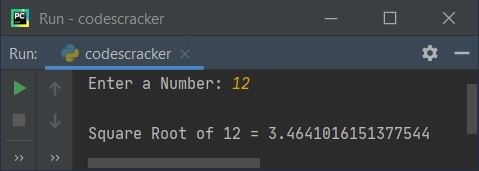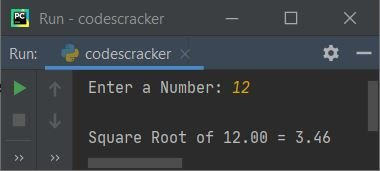# Python Program to Find Square Root of a Number

This article is created to cover some programs in Python, that find and print square root of a number entered by user at run-time. Here are the list of approaches used:

• Find square root of a number using ** operator
• Using math.sqrt() inbuilt function
• Using math.pow()
• Using user-defined function
• Using class

## Find Square Root of a Number using **

To find square root of any number given by user in Python, you have to ask from user to enter a number, then find and print its square root like shown in the program given below. The question is, write a Python program to find square root of a number. Here is its answer:

```print("Enter a Number: ")
num = int(input())

squareroot = num ** 0.5

print("\nSquare Root =", squareroot)```

Here is the initial output produced by this Python program:Now enter any number say 25 and press `ENTER` key to find and print its square root like shown in the snapshot given below:Note - The a ** b returns ab. So num ** 0.5 refers to num0.5 (that equals num1/2).

## Find Square Root using math.sqrt() Inbuilt Function

This program uses math.sqrt() to find square root. This method is defined in math module, therefore before using it, I've to import math module:

```import math

print("Enter a Number: ", end="")
try:
num = int(input())
res = math.sqrt(num)
print("\nSquare Root of " +str(num)+ " = " +str(res))
except ValueError:
print("\nInvalid Input!")```

Here is its sample run with user input 12:To format value upto only two decimal places, then replace the following statement (from above program):

`print("\nSquare Root of " +str(num)+ " = " +str(res))`

with the statement given below:

`print("\nSquare Root of %0.2f = %0.2f" %(num, res))`

now the output looks like with same user input, that is 12:The str() method is used to convert any type of value into a string type value.

## Find Square Root using math.pow()

This program uses pow() method to find square root. The math.pow(a, b) returns the value of ab. So math.pow(num, 0.5) refers to num0.5.

```import math

print("Enter a Number: ", end="")
num = int(input())

res = math.pow(num, 0.5)
print("\nSquare Root of %0.2f = %0.2f" %(num, res))```

## Find Square Root using User-defined Function

This program is created using a user-defined function named sqrt(), that returns the square root of a number passed as its argument. Therefore using res = sqrt(num), the square root of num gets initialized to res.

```def sqrt(n):
return n ** 0.5

print("Enter a Number: ", end="")
num = int(input())

res = sqrt(num)
print("\nSquare Root of %0.2f = %0.2f" %(num, res))```

## Find Square Root using Class

This is the last program created using class. Class is an object-oriented feature of Python. Function inside a class is called as member function. Therefore to access it, an object of class is required. So I've created an object named obj of class CodesCracker to access its member function using dot (.) operator.

```class CodesCracker:
def sqrt(self, n):
return n ** 0.5

print("Enter a Number: ", end="")
num = int(input())

obj = CodesCracker()
print("\nSquare Root of %0.2f = %0.2f" %(num, obj.sqrt(num)))```

Python Online Test

« Previous Program Next Program »